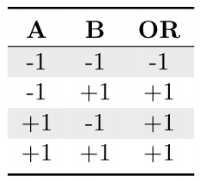### Random Thoughts on Linear Classifiers I

One can define machine learning (ML) as programs that learn and improve with the use of the experience at some task using a measure of the performance. We can classify ML techniques basing on the desired outcome of the algorithm. There are, in the classic taxonomy, three main types of learning: (1) supervised learning, where an expert provides feedback in the learning process, (2) unsupervised learning, where there is no teacher or expert when the learning process is running, and (3) reinforcement learning, where the program learns interacting with the environment.

Of all these, we will talk about the first one. Supervised learning is a ML method for extracting a model from training data. These data consist of a set (called examples) of input attributes (sometimes called features) and the desired output. As commented before, the main characteristic of supervised learning is that the program needs an expert or teacher that provides feedback in the learning process. Typically, the supervised learner uses the provided output (called label, class or category) of the training data as a guidance.

We can further classify supervised learning depending on the type of the output attributes as (1) data classification and (2) data regression. In data classification, the goal is to find a model that predicts the class (that is, the output attribute) of new input instances. In data regression, the goal is to find a function that predicts the output value of new input instances.

In this entry we will see one of the easiest classifiers: the linear classifiers. To simplify the text, we will assume that we have two classes, formally known as positive class {+} and negative class {-} (this is called binary classification since we have only two classes). To understand linear classifiers, some theoretical notions are first given. We will finish with a practical approach coding a perceptron, the first neural network unit.

Formally, binary classification is performed by, having the input xj, where xj is a vector consisting in the input features, assigning this input xj to the positive class {+} if a given linear function f(x) is greater or equal to zero, and otherwise assigning it to the negative class {-}. The key of linear classifiers is the linear function. In the case of a perceptron, this function is defined as a linear combination of its inputs using a weight vector w and a bias weight b in the following manner: f(x) = w · x + b, where w · x is the dot product of the vectors w and x (that is, w1·x1 + w2 · x2 + … + wn · xn).

Linear classifiers split the input space in two parts by the hyperplane defined by the equation w · x + b = 0, and the vector w defines a direction perpendicular to the plane, while varying the value of b moves this hyperplane parallel to itself.

This may sound tricky, but the idea is very simple. Let's do the classical example: the OR problem. In the OR problem we want that our classifier learns the logical OR function by training with the following dataset:We want a hyperplane (that is, a straight line) that separates the two classes and allow us to classify in which class new input data will fit.

We have two input features (A and B) and one output label (OR) with two classes {-1} and {+1}. Our problem is how to learn that separating hyperplane from training data. The perceptron solves this with an iterative algorithm that modifies the weights according to the misclassifications occurred during the training.

In the next entry we will discuss the code of the perceptron.